# 概率图模型

Posted by MaggicQ on September 18, 2018

# 概率图模型

• $V$ 表示节点即随机变量（放在此处的，可以是一个token或者一个label），具体地，用 $Y = (y_{1}, {\cdots}, y_{n} )$ 为随机变量建模，注意 $Y$ 现在是代表了一批随机变量（想象对应一条sequence，包含了很多的token）， $P(Y)$ 为这些随机变量的分布；
• $E$ 表示边即概率依赖关系。具体咋理解，还是要在后面结合HMM或CRF的graph具体解释。

## 基本概念

• 有向图模型（如贝叶斯网络）适合为有单向依赖的数据建模
• 无向图模型（如马尔可夫网络）适合实体之间相互依赖的建模

## 隐马尔可夫模型（Hidden Markov Model，HMM）

### 三个要素

• $A$ ，状态转移概率矩阵， $A= [a_{ij}]_{N \times N}$ （N为隐藏状态集元素个数），其中 $a_{ij} = P(i_{t+1} = q_j |i_{t} = q_i)$，表示在时刻t处于状态$q_i$条件下在时刻$t+1$转移到状态$q_j$的概率。
• $B$ ，观测概率矩阵，$B=\left[b_{j}(k)\right]_{N \times M}$（N为隐藏状态集元素个数，M为观测集元素个数），其中 $b_{j}(k)=P\left(o_{t}=v_{k} | i_{t}=q_{j}\right), \quad k=1,2, \cdots, M ; j=1,2, \cdots, N$ 是在时刻t处于状态$q_j$的条件下生成观测$v_k$的概率。
• $π$ ，初始状态分布，$\pi = (\pi_i)$其中$\pi_i = P(i_1 = q_i), i = 1,2, \cdots, N$是时刻$t=1$处于状态$q_i$的概率。

### 两个基本假设

• 齐次马尔可夫性假设，即假设隐藏的马尔可夫链在任意时刻$t$的状态只依赖于其前一时刻的状态，与其他时刻的状态及观测无关，也与时刻$t$无关。
• 观测独立性假设，即假设任意时刻的观测只依赖于该时刻的马尔可夫链的状态，与其他观测及状态无关。

### 三个基本问题

• 概率计算问题，给定模型$\lambda = (A, B, \pi)$和观测序列$O = (o_1, o_2, …, o_T)$，计算在模型$\lambda$下观测序列$O$出现的概率$P(O | \lambda)$
• 学习问题。已知观测序列$O=(o_1,o_2,…,o_T)$，估计模型$\lambda = (A, B, \pi)$参数，使得在该模型下观测序列概率$P(O| \lambda)$最大，即用极大似然估计的方法估计模型参数
• 预测问题，已知模型$\lambda = (A, B, \pi)$和观测序列$O = (o_1, o_2, …, o_T)$，求对给定观测序列条件概率$P(I | O)$最大的状态序列$I$。即给定观测序列和模型参数，求最有可能的对应的状态序列

#### 概率计算算法

• 直接计算法，按概率公式直接计算$P(O | \lambda)=\sum_{I} P(O | I, \lambda) P(I | \lambda)$， 这样做的话计算量太大，不可行。复杂度$O(TN^T)$。

• 前向算法：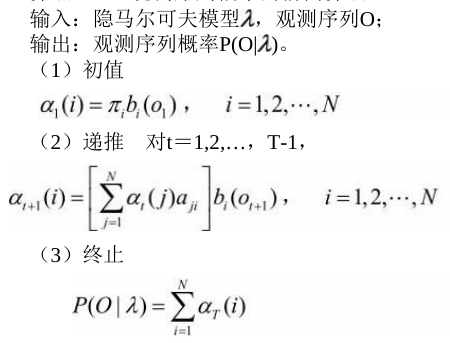其中$\alpha_t(j)$是到时刻$t$观测到$o_1, o_2, …, o_t$并在时刻$t$处于状态$q_j$的前向概率，那么乘积$\alpha_t(j)a_{ji}$就是到时刻$t$观测到$o_1, o_2, …, o_t$并在时刻$t$处于状态$q_j$而在时刻$t+1$到达状态$q_i$的联合概率。所以$\left[\sum_{j=1}^{N} \alpha_{t}(j) a_{j i}\right]$就表示结果就是到时刻$t$观测为$o_1,o_2,…,o_t$并在时刻$t+1$处于状态$q_i$的联合概率。下面这张图帮助理解前向概率的递推公式：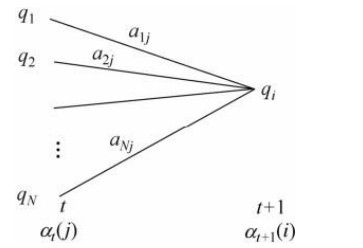前向算法减少计算量的原因在于每一次计算直接引用前一个时刻的计算结果，避免重复计算。它的复杂度是$O(N^2T)$。

• 后向算法，与前向算法类似：

输入:隐马尔可夫模型$\lambda$，观测序列$O$; 输出:观测序列概率$P(O| \lambda )$。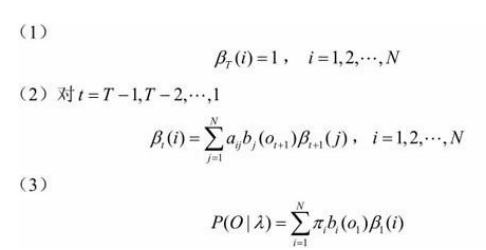后向概率$\beta_t(i)$的定义：在时刻$t$状态为$q_i$条件下时刻$t+1$之后的观测序列为$o_{t+1},o_{t+2},…,o_{T}$的概率。第一步初始化，第二步递推，第三步就可以求结果了。其中递推公式：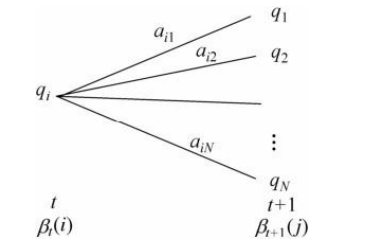算法的复杂度也是$O(N^2T)$

#### 学习算法

##### 监督学习方法

• 设样本中时刻$t$处于状态$i$时刻$t+1$转移到状态j的频数为$A_{ij}$，那么转移概率$a_{ij}$的估计：
• 设样本中状态为j并观测为k的频数是$B_{jk}$，那么状态为j观测为k的概率$b_j(k)$的估计是:
• 初始状态概率 $\pi_i$的估计$\hat{\pi_i}$为S个样本中初始状态为$q_i$的频率

##### Baum-Welch算法(EM算法)#### 预测算法

##### 维特比算法

• 在时刻t状态为i的所有单个路径$(i_1, i_2, …. , i_t)$中的概率最大值为 $\delta_t(i)$，递推公式：
• 定义在时刻t状态为i的所有单个路径$(i_1, i_2, …. , i_t)$中概率最大的路径的第$t-1$个结点为：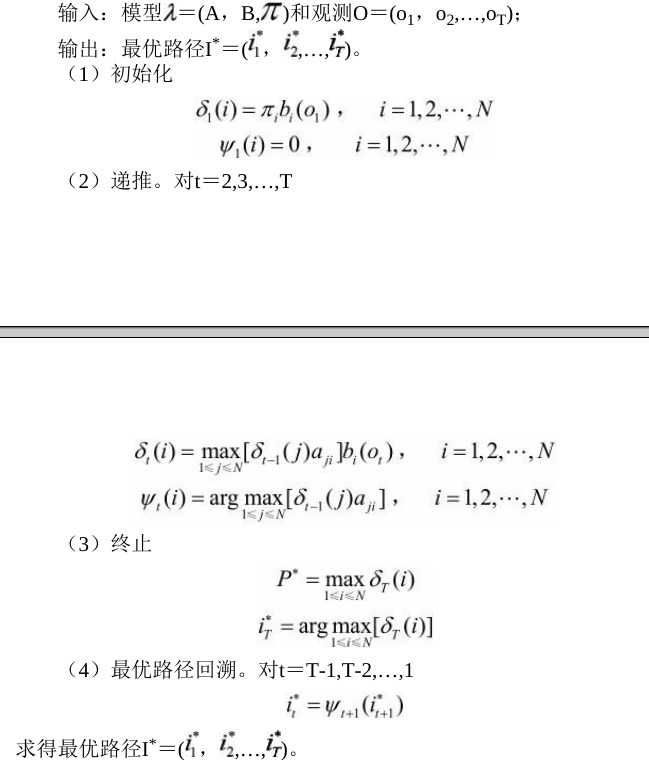## 最大熵马尔可夫模型

HMM中，观测节点 $o_{i}$ 依赖隐藏状态节点 $i_{i}$ ,也就意味着我的观测节点只依赖当前时刻的隐藏状态。但在更多的实际场景下，观测序列是需要很多的特征来刻画的，比如说，我在做NER时，我的标注 $i_{i}$ 不仅跟当前状态 $o_{i}$ 相关，而且还跟前后标注 $o_{j}(j \neq i)$ 相关，比如字母大小写、词性等等。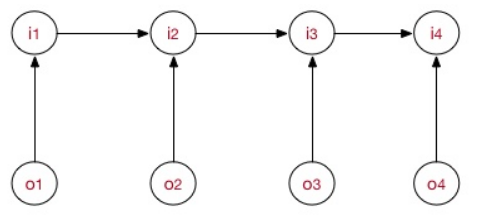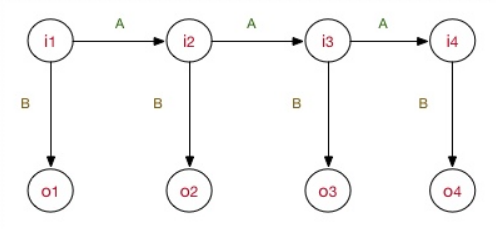MEMM模型的工作流程也包括了学习训练问题、预测问题、序列求概率问题。

## CRF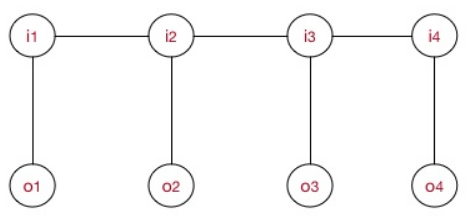• 下标i表示我当前所在的节点（token）位置。
• 下标k表示我这是第几个特征函数，并且每个特征函数都附属一个权重 $\lambda_{k}$ ，也就是这么回事，每个团里面，我将为 $token_{i}$ 构造M个特征，每个特征执行一定的限定作用，然后建模时我再为每个特征函数加权求和。
• $Z(O)$ 是用来归一化的，这是为了将其转化为概率值。
• $P(I|O)$ 这个表示什么？具体地，表示了在给定的一条观测序列 $O=(o_{1},\cdots, o_{i})$ 条件下，我用CRF所求出来的隐状态序列 $I=(i_{1},\cdots, i_{i})$ 的概率，注意，这里的I是一条序列，有多个元素（一组随机变量），而至于观测序列 $O=(o_{1},\cdots, o_{i})$ ，它可以是一整个训练语料的所有的观测序列；也可以是在inference阶段的一句sample，比如说对于序列标注问题，我对一条sample进行预测，可能能得到 $P_{j}(I | O)（j=1,…,J）$J条隐状态I，但我肯定最终选的是最优概率的那条（by viterbi）。这一点希望你能理解。

• $t_{j}(O,I_{i-1},I_{i},i)$ 为$i$处的转移特征，对应权重 $\lambda_{j}$ ，每个 $token_{i}$ 都有$J$个特征，转移特征针对的是前后token之间的限定。举个例子：
• $s_{l}(O,I_{i},i)$为处$i$的状态特征，对应权重$\mu_l$，每个$token_i$都有$L$个特征，举个例子：

log-linear models take the following form: $P(y|x;\omega) = \frac{ exp(\omega·\phi(x,y)) }{ \sum_{y^{‘}\in Y }exp(\omega·\phi(x,y^{‘})) }$

CRF的学习训练过程

## 总结

NB在sequence建模下拓展到了HMM；LR在sequence建模下拓展到了CRF。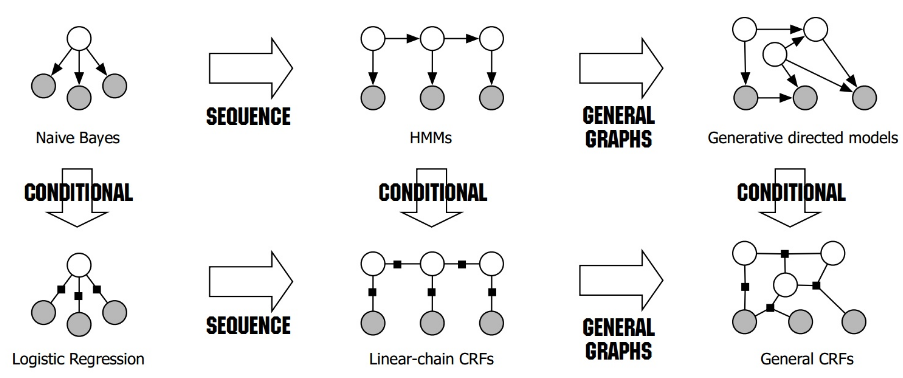1. HMM -> MEMM： HMM模型中存在两个假设：一是输出观察值之间严格独立，二是状态的转移过程中当前状态只与前一状态有关。但实际上序列标注问题不仅和单个词相关，而且和观察序列的长度，单词的上下文，等等相关。MEMM解决了HMM输出独立性假设的问题。因为HMM只限定在了观测与状态之间的依赖，而MEMM引入自定义特征函数，不仅可以表达观测之间的依赖，还可表示当前观测与前后多个状态之间的复杂依赖。
2. MEMM -> CRF:
1. CRF不仅解决了HMM输出独立性假设的问题，还解决了MEMM的标注偏置问题，MEMM容易陷入局部最优是因为只在局部做归一化，而CRF统计了全局概率，在做归一化时考虑了数据在全局的分布，而不是仅仅在局部归一化，这样就解决了MEMM中的标记偏置的问题。使得序列标注的解码变得最优解。
2. HMM、MEMM属于有向图，所以考虑了x与y的影响，但没讲x当做整体考虑进去（这点问题应该只有HMM）。CRF属于无向图，没有这种依赖性，克服此问题。